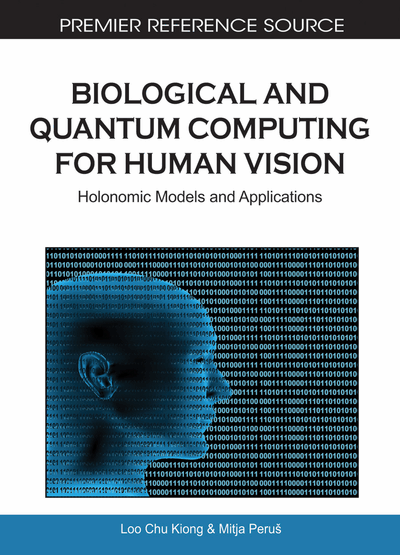# Quantum Neural Information Processing

DOI: 10.4018/978-1-61520-785-5.ch009
OnDemand:
(Individual Chapters)
Available
\$37.50
No Current Special Offers

## Chapter Preview

Memory is connected to the ‘wavefunction’ by the probability distribution ∣Ψ∣2 which is peaked 2 at the singularity [...] in the Schrodinger equation. In full analogy with the classical system [ANN (note by M.P.)], this singularity occurs at the same location in the activity space as the resting point of the classical dynamical trajectory [...]. [...]

An additional similarity is that a certain measurement time is required to gain a precise value for the location of the attractor. In the classical system this consists of waiting for the motion of the pseudo ”particle” [i.e., ANN state (note by M.P.)] to exponentially slow to the point where it can reasonably be considered to have stopped [i.e., at the bottom of the potential-well corresponding to the attractor (note by M.P.)]. Likewise, the quantum system requires a sufficient amount of time to perform enough measurements to determine the average probability distribution.

Bonnell & Papini (1997) calculated wave-functionswhich encode memory-attractors in their net for the cases of symmetric weights (Jij= Jij) and small-magnitude asymmetric weights. The approximate solution of the Schrodinger equation in the first case are Gaussian wave-functions centred on the activity-point () in a net of two neurons (with activities q1 and q2):

(9.1)

All quantum information systems (AuxLit 22-25) promise miniaturization of PDP implementation, and much greater speed, efficiency and computational capacity. The main source of significant improvements in performance of the quantum associative net and similar models (Wang et al., 1998) is, like in HNeT (as shown in Sutherland, 1990, 1994), the use of quantum phase φ, hidden in, for encoding and processing of information (details in Perus & Dey, 2000).

See Chapter 7 for explicit mathematical presentation of quantum-holographic realization of my Quantum Associative Network model for the special case of image recognition using quantum waves.

## Complete Chapter List

Search this Book:
Reset